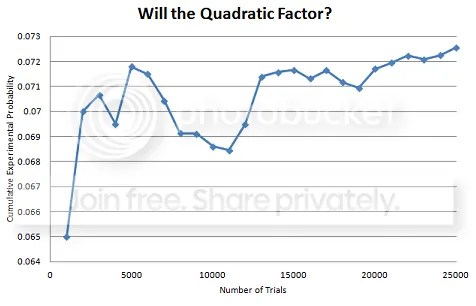Categories

# What’s the Probability That Quadratic Will Factor?

A comment from my post last week about the need for factoring led me to re-visit a question I have posed to classes before, but never allowed to move beyond the “gee, that’s interesting” stage.

Given a polynomial in standard form, with random non-zero* integer parameters a, b and c, what is the probability that the polynomial will factor?

I’ve pursued this question with classes before by writing a polynomial on the board, with blanks or boxes in the a-b-c positions.  Sometimes, I would take “random” shout-outs from the class to fill in the boxes.  With another class, the randint function on a TI calculator was used to generate our abc’s.  The point was to demonstrate that a large majority of quadratics are not factorable, and that despite the nice, rigged, problems we encounter in textbooks, we should spend far more time considering what to do with the messy ones.  But I’d never put pencil to paper and thought about the theoretical probability.

After my post on factoring last week, Jim Doherty mentioned a speaker he had encountered find an experimental probability that a quadratic would factor, and cited 7%.  That number seemed reasonable to me, but perhaps a bit on the high side.  I set up an Excel document to generate three non-zero integers (more on this later), and rigged a system to check for perfect-square discriminants.  I recorded experimental results, in groups of 1000 trials, and kept a running total.After 25,000 trials, I found that 7.26% of the quadratics would factor.

*While this endeavor started off innocently and quickly enough, I had to start over after I realized my Excel document allowed for zeroes.  It took a little logical Excel rigging to exclude them.

So, there must be a theoretical probability out there someplace?  Anyone know how to do it?## By Bob Lochel

HS Math Teacher. Hatboro-Horsham School District, Horsham, PA.

## 4 replies on “What’s the Probability That Quadratic Will Factor?”mrsiderersays:

I am surprised that the percentage is even that high. Please let me know if you pursue this, and how it works out. How large did you allow the a, b and c to be?

a,b,c were allowed to each go up to 20. It would be a fairly easy task to allow them to go higher and perform a bigger simulation, but I suspect there must be a more direct proof.Joel Tsays:

Allowing the coefficients to be integers as large as possible, the probability is exactly zero.
Here’s why :Look at the discriminant, square root of b squared minus 4ac.
If that number is rational then the quadratic can factor (over the integers).
If the discriminant is irrational then the quadratic can’t factor.
But the number of rationals is countable (aleph-null) while the irrationals are uncountable (a “larger” infinity).
So the ratio of factorable to unfactorable quadratics is zero.Robert Gilpatricsays:

If coefficients a, b, and c are integers from -20 to 20, there are 64000 possible quadratics of which 4628 are factorable. (0.0723125)